### Case-Level Residuals, Outliers, Leverage Observations, and Residual Diagnostics

#### Residual M-Distances and Outlier Detection

In structural equation modeling, residual analysis has been developed traditionally for the elements in the moment matrices such as mean vector and covariance matrix. See the section Residuals in the Moment Matrices for a detailed description of this type of residual. In fact, almost all fit indices in the field were developed more or less based on measuring the overall magnitude of the residuals in the moment matrices. See the section Assessment of Fit for examples of fit indices such as standardized root mean square residual (SRMR), adjusted goodness-of-fit index (AGFI), and so on.

However, recent research advances make it possible to study case-level or observational-level residuals. Although case-level residuals have not yet been used to assess overall model fit, they are quite useful as model diagnostic tools. This section describes how case-level residuals are computed and applied to the detection of outliers, the identification of leverage observations, and various residual diagnostics.

The main difficulty of case-level residual analysis in general structural equation modeling is the presence of latent variables in the model. Another difficulty is the involvement of multivariate responses. Both are nonissues in multiple regression analysis because there is no latent variable and there is only a single response variable. Hence, a good starting point for this section is to explain how PROC CALIS handles these two issues in general structural equation modeling. The residual diagnostic techniques described in the following draw heavily from Yuan and Hayashi (2010).

Latent variables in models are not observed, and their values must be predicted from the data. Although the indeterminacy of factor scores is a well-known issue, it does not mean that factor scores cannot be reasonably derived or predicted from the observed data. One of the factor score estimation methods is Bartlett’s method. Suppose for a factor model that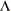represents a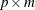factor matrix and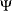represents a
error covariance matrix, where p is the number of manifest variables and m is the number of factors. Bartlett’s factor scores are defined by the formula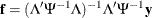whererepresents an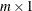random vector of factors andrepresents a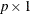random vector of manifest variables. Yuan and Hayashi (2010) generalize Bartlett’s formulas to a certain class of structural equation models. Essentially, they provide formulas that the quantitiesandare computable functions from the parameter estimates of the structural equation models. PROC CALIS adopts their formulas. With the estimation of factor scores, residuals in structural equation models can be computed as if all values of factors are present. Hence, case-level residual analysis becomes possible.

With the possibility of a multivariate residual vector in structural equation models, a summary measure for the overall magnitude of the residuals in observations is needed. Yuan and Hayashi (2010) consider the Mahalanobis distance (M-distance) of case-level residuals. For observation i with residual vector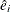of dimension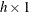, the residual M-distance of observation i is defined as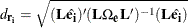where(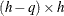) is a matrix that reducesto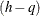independent components, q is the number of independent factors to be estimated in the structural equation model, and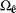is the covariance matrix of. The reduction of the residual vector into independent components is necessary when the number of factors q is not zero in the model. For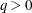,is not invertible and cannot be used in computing the M-distances for residuals. Hence, the covariance matrix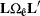of independent components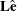is used instead. In practice,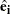andare evaluated at the estimated parameter values.can be computed afteris estimated.

Because the residual M-distance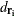is nonnegative and is a distance measure, the interpretation ofis straightforward—the larger it is, the farther away observation i is from the origin of the residuals. Therefore, the residual M-distancecan be used to detect outliers. The criterion for defining outliers is based on a selection of an-level for the residual M-distance distribution. Under the multivariate normality distribution of the residuals, Yuan and Hayashi (2010) find thatis distributed as a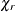variate (that is, the square root of the corresponding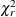variate), where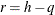is the degrees of freedom of the distribution. Therefore, a probability-based criterion for outlier detection can be used. An observation is an outlier according to the-level criterion if its residual M-distanceis located within the upper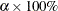region of thedistribution. Mathematically, an observation withsatisfying the following criterion is an outlier if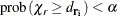The larger the, the more liberal the criterion for detecting outliers. By default, PROC CALIS setsto 0.01. You can override this value by using the ALPHAOUT= option.

#### Leverage Observations

Leverage observations are those with large M-distances in the exogenous variables of the model. For example, in the context of a confirmatory factor model, Yuan and Hayashi (2010) define the M-distance of exogenous latent factorsby the formula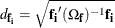where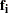is the Bartlett’s factor scores for observation i and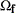is the covariance matrix of the factor scores. See Yuan and Hayashi (2010) for the formulas forand. PROC CALIS uses a more general formula to compute the M-distance of exogenous observed variables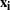and latent variablesin structural equation models. That is, with exogenous variables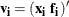, the leverage M-distance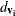is computed as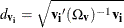where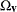is the covariance matrix of the exogenous variables. Leverage observations are those judged to have large leverage M-distances.

As with outlier detection, you need to set a criterion for declaring leverage observations. Specifically, an observation withsatisfying the following criterion is a leverage observation (or leverage point):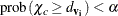where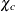is the square root of the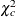variate, with c being the dimension of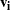. The larger the, the more liberal the criterion for declaring leverage observations. The defaultvalue is 0.01. You can override this value by using the ALPHALEV= option.

Unlike the outliers that are usually judged as undesirable in models, leverage observations might or might not be bad for model estimation. Simply stated, a leverage observation is bad if it is also an outlier according to its residual M-distance value. Otherwise, it is a good leverage observation and should not be removed in model estimation.

#### Residual Diagnostics

Case-level residuals analysis can be very useful in detecting outliers, anomalies in residual distributions, and nonlinear functional relationships. All these techniques are graphical in nature and can be found in most textbooks in regression analysis (see, for example, Belsley, Kuh, and Welsch 1980). PROC CALIS provides these graphical analyses in the context of structural equation modeling. However, because PROC CALIS deals with multivariate responses and predictors in general, in some residual analysis the residuals are measured in terms of M-distances, which are always positive. PROC CALIS can produce the following graphical plots (with their ODS graph names in parentheses) for residual diagnostics:

• Residual histogram (CaseResidualHistogram): the distribution of residual M-distances is shown as a histogram. The residual M-distances are theoretically distributed as a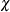-variate. Use the PLOTS=CRESHIST option to request this plot.

• Residual by leverage plot (ResidualByLeverage): the observations are plotted in a two-dimensional space according to their M-distances for residuals and leverages. Criteria for classifying outliers and leverage observations are shown in the plot so that outliers and leverage observations are located in well-defined regions of the two-dimensional space. Use the PLOTS=RESBYLEV option to request this plot.

• Residual by predicted values (ResidualByPredicted): the residuals (not the M-distances) of a dependent observed variable are plotted against the predicted values. This is also called the residual on fit plot. If the relationship of the dependent variable to the predictors is truly linear in the model, the residuals should be randomly dispersed in the plot. If the residuals show some systematic pattern with the predicted values, unexplained nonlinear relationships of the dependent observed variable with other variables or heteroscedasticity of error variance might exist. Use the PLOTS=RESBYPRED option to request this plot.

• Residual by quantile plot or Q-Q plot (ResidualByQuantile): the residual M-distances are plotted against their theoretical quantiles. Observations that depart significantly from the theoretical line (with slope=1) are problematic observations. Use the PLOTS=QQPLOT option to request this plot.

• Residual percentile by theoretical percentile plot or P-P plot (ResPercentileByExpPercentile): the observed residual M-distance percentiles are plotted against the theoretical percentiles. Observations that depart significantly from the theoretical line (with slope=1) are problematic observations. Use the PLOTS=PPPLOT option to request this plot.

#### Residual Diagnostics with Robust Estimation

With the presence of multiple outliers in a data set, estimation might suffer from the so-called masking effect. This problem refers to the fact that some prominent outliers in the data might have biased the estimation so that usual residual diagnostic tools might fail to detect some less prominent outliers. As a result, subsequent analyses that are based on the data set with outliers removed might actually not be free from the effects of the outliers.

Robust estimation deals with the outliers differently. Instead of relying on diagnostic tools for detection and removal of outliers, robust methods downweight the outliers during the estimation so that the undesirable outlier effect on estimation is minimized effectively. No outliers need to be removed during or after the robust estimation. Hence, the masking effect is not an issue. In addition, with the use of robust estimation, residual diagnostics for the purpose of outlier removal become a redundant concept. In such a scenario, outlier diagnostics are more important for the purpose of identification than for removal. You can use the ROBUST=RES option (or the default ROBUST option) to downweight the outliers during model estimation.

Similarly, detecting leverage observations might also suffer from the masking effect. That is, some leverage observations might not be detected if the diagnostic results are based on model estimation that includes the leverage observations themselves. Unfortunately, the ROBUST=RES option downweights only the residual outliers and does not downweight the leverage observations during estimation. However, the ROBUST=SAT option does downweight outlying observations in all variable dimensions, and hence it could be used if the masking effect of leverage observations is a concern.

Following the recommendation by Yuan and Hayashi (2010), PROC CALIS uses an eclectic approach to compute the residual and leverage M-distances. That is, when a robust estimation is requested (using either the ROBUST=SAT or ROBUST=RES option) with case-level residual analysis (using the RESIDUAL option), the residual M-distances are computed from the robust estimation results that downweight the model outliers only (that is, the procedure invoked by the ROBUST=RES(E) or RES(F) option); but the leverage M-distances are computed from the robust estimation results that downweight the outlying observations in all variable dimensions (that is, the procedure invoked by the ROBUST=SAT option). Consequently, both the residual M-distances and the leverage M-distances calculated from this eclectic approach are free from the respective masking effects—and this is true for any robust estimation methods implemented in PROC CALIS.

It is important to note that this eclectic approach applies only to the computations of the residual and leverage M-distances. The parameter estimates, fit statistics, and the case-level residuals that are displayed in the residual on fit plots are still obtained from the specific robust method requested. Table 27.6 summarizes the robust methods that PROC CALIS uses to compute the parameter estimates, fit statistics, case-level residuals, and residual and leverage M-distances with different robust options.

Table 27.6: Robust Methods for the Computations of Various Results

Robust Option Specified

ROBUST=SAT

ROBUST=RES(E)

ROBUST=RES(F)

Estimates

ROBUST=SAT

ROBUST=RES(E)

ROBUST=RES(F)

Model fit

ROBUST=SAT

ROBUST=RES(E)

ROBUST=RES(F)

Case-level residuals

ROBUST=SAT

ROBUST=RES(E)

ROBUST=RES(F)

Residual M-distances

ROBUST=RES(E)

ROBUST=RES(E)

ROBUST=RES(F)

Leverage M-distances

ROBUST=SAT

ROBUST=SAT

ROBUST=SAT

One important implication from this table is that ROBUST=SAT and ROBUST=RES(E) lead to the same residual and leverage diagnostic results, which are all free from masking effects.

In conclusion, even though outlier removal is unnecessary with robust estimation, case-level residual diagnostics are still useful for identifying outliers and leverage observations. Needless to say, other residual diagnostics such as residual M-distance distribution, Q-Q plots, P-P plots, and residual on fit plots are useful whether you use robust estimation or not.